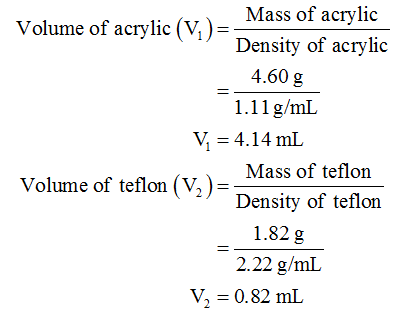# Say you glued two samples together; a 4.60 gg  sample of acrylic with a density of 1.11 g/mLg/mL  and a 1.82 gg  sample of Teflon (d = 2.22 g/mL). What is the density of the combined sample? Be careful here and remember the difference between intensive and extensive properties.

Question
43 views

Say you glued two samples together; a 4.60 gg  sample of acrylic with a density of 1.11 g/mLg/mL  and a 1.82 gg  sample of Teflon (d = 2.22 g/mL). What is the density of the combined sample? Be careful here and remember the difference between intensive and extensive properties.

check_circle

Step 1

It is given that:

Mass of acrylic sample (m1) = 4.60 g

Density of acrylic sample = 1.11 g/mL

Mass of sample of Teflon (m2) = 1.82 g

Density of sample of Teflon = 2.22 g/mL

Step 2

The properties which depend on the amount of matter present in it are called extensive properties whereas the properties which do not depend on the amount of matter present in it are called intensive property.

For example Mass, volume, etc. are extensive property whereas enthalpy, density is intensive properties.

The ratio for two mass-dependent that is extensive property is always an intensive property.

Step 3

Density is defined as mass per unit volume (Density = Mass/volume). Thus, the volume can be calculated using density expression ( V = mass/density ).

Therefore, the volume of both acrylic and Teflon will be calculated as follows:...

### Want to see the full answer?

See Solution

#### Want to see this answer and more?

Solutions are written by subject experts who are available 24/7. Questions are typically answered within 1 hour.*

See Solution
*Response times may vary by subject and question.
Tagged in

### Chemistry